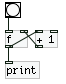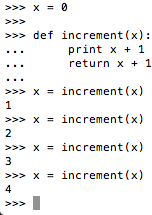• ### How can I replicate a simple counter, like [f]x[+], in python?

Hi forum!

How would I do this in python?So far I have this:

`````` x = 0

def increment(x):
print x + 1
return x + 1

x = increment(x)
``````

But every time I run this function I feel that x just disappears or resets itself. Any guidance would be much appreciated.

• | Posts 12 | Views 4288
• Probably a silly question, but you are not by any chance always including the first line where you reset the variable `x` to 0, are you?

• @gsagostinho I am! And I know that sounds really dumb because it forces x to 0, but I can't figure out how to avoid this error otherwise: `NameError: name 'x' is not defined`

• Well, you do have to give the variable a starting value at some point, but you don't have to use that part of the code ever again. Have a look:

``````    >>> def increment(x):
...     print x + 1
...     return x + 1
...
>>> x = 0
>>> x = increment(x)
1
>>> x = increment(x)
2
>>> x = increment(x)
3
>>> x = increment(x)
4
>>> x = increment(x)
5
``````

What exactly are you trying to accomplish?

Cheers,
Gilberto

EDIT: above I am dynamically typing the code and getting the results, hence the `>>>` and `...` before the programming commands.

• @gsagostinho By dynamically typing, do you mean just copy and pasting the `x = increment(x)` line?

• No, I mean something else. Python works in two ways: you can either create a python file (.py) and execute it, or you can run the Python Interpreter, which evaluates every single command as you type. Both obviously produce the same results and are just two different ways of accomplishing the same thing.

• @gsagostinho Ok so before I was just executing the whole .py program. Using python in the shell, and just copy and pasting the `x = increment(x)` line, I'm getting the results I was hoping for, so thank you for that!Awesome!

Here's what I am trying to do: As a programming exercise for myself, I just want to recreate that simple pd counter above. I already coded a little widget in Tkinter that gives me a button and label, and every time I click that button with a mouse I want to increment a counter. I know how to "capture" the event of clicking a button, but I don't know how to make it just execute that one `x = increment(x)` line of code. I'll see if I can post the rest of my code here.

• Cool, glad to hear it works!

But note that if you save the following code to a .py file (let's say `test.py`):

``````    def increment(x):
print x + 1
return x + 1

x = 0

x = increment(x)
x = increment(x)
x = increment(x)
x = increment(x)
``````

And then execute it with `python ./test.py`, you also get the expected result:

``````gilberto@gilberto-Inspiron-7520 ~/\$ python ./test.py
1
2
3
4
``````

Cheers!
Gilberto

• For anyone curious, here's what I eventually came up with:

`````` import Tkinter as tk
from Tkinter import *

root=tk.Tk()
root.title("button")

var=StringVar()
var.set('bang')

x = 0

def increment():
global x
x += 1
print x

def ButtonClick():
increment()

def ButtonClick2():
print '--------------------\n', 'You closed me :('
root.destroy()

button = tk.Button(root, textvariable=var, command=ButtonClick).grid(row='0', column='0')
button2 = tk.Button(root, text="Close", command=ButtonClick2).grid(row='0', column='1')

root.mainloop()``````
• BTW for other beginning users out there like myself, to run this here's what you do:

1. Save it somewhere as a .py file
2. Run in python via command line (you'll have to google this depending on your OS)
3. The results of the "bang" are posted to the command line
| Posts 12 | Views 4288
Internal error.

Oops! Looks like something went wrong!